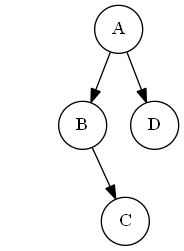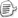N → a ( N, N )N → εclass Node { public Node LeftChild { get; private set; } public Node RightChild { get; private set; } public char Label { get; private set; } public Node(char label, Node left, Node right) { Label = label; LeftChild = left; RightChild = right; } } 

1. 使用一个索引来记录当前扫描的位置。通常将它做成一个整数字段。
2. 为每个非终结符编写一个方法。
3. 如果一个非终结符有超过一个的产生式，则在这个方法中对采用哪个产生式进行分支预测
4. 处理单一产生式时，遇到正确终结符则将第一步创建的扫描索引位置向前移动；如遇到非终结符则调用第二步中创建的相应方法。
5. 如果需要产生解析的结果（比如本例中的二叉树），在方法返回之前将它构造出来。

 class BinaryTreeParser { private string m_inputString; private int m_index; //初始化输入字符串和索引的构造函数，略 Node ParseNode() { } } 

1. 如果超前查看遇到英文字母，预测分支N → a(N, N)
2. 如果超前查看遇到逗号、右括号预测分支N → ε

 Node ParseNode() { int lookAheadIndex = m_index; char lookAheadChar = m_inputString[lookAheadIndex]; if (Char.IsLetter(lookAheadChar)) { //采用N → a(N, N)继续分析 } else if (lookAheadChar == ',' || lookAheadChar == ')' ) { //采用N → ε继续分析 } else { throw new Exception("语法错误"); } } 

 Node ParseNode() { int lookAheadIndex = m_index; char lookAheadChar = m_inputString[lookAheadIndex]; if (Char.IsLetter(lookAheadChar)) { //采用N → a(N, N)继续分析 char label = m_inputString[m_index++]; //解析字母 m_index++; //解析左括号，因为不需要使用它的值，所以直接跳过 Node left = ParseNode(); //非终结符N，递归调用 m_index++; //解析逗号，跳过 Node right = ParseNode(); //非终结符N，递归调用 m_index++; //解析右括号，跳过 return new Node(label, left, right); } else if (lookAheadChar == ',' || lookAheadChar == ')') { //采用N → ε继续分析 //无需消耗输入字符，直接返回null return null; } else { throw new Exception("语法错误"); } } 

 F → idF → ( E )E → F * FE → F / F

 F → idF → ( E )G → * FG → / FE → FG

 F → idE → E + FE → F

 F → idE → FGG → + FGG → εposted on 2011-06-21 00:22  装配脑袋  阅读(37871)  评论(25编辑  收藏  举报